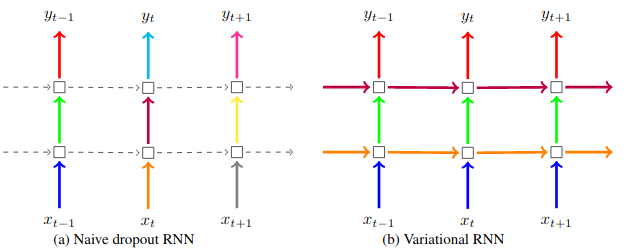# Value Error : can't optimize a non-leaf Tensor

Hey I am new to forum. Please tell me if this is the correct thread to post it , if not so I can change the category.

My question is as follows

Pytorch Doesn’t Support Variational Dropout in LSTM cell. So I have borrowed one from fastAI implementation.Essentially Variational Dropout will be using same dropout masks at every time step.I can modify it from WeightDropout Module implemented in fastai library. So I took the source code from there . But there is Slight Glitch.

class WeightDropout(Module):
"A module that warps another layer in which some weights will be replaced by 0 during training."

def __init__(self, module:nn.Module, weight_p:float, layer_names:Collection[str]=['weight_hh_l0']):
self.module,self.weight_p,self.layer_names = module,weight_p,layer_names
self.idxs = [] if hasattr(self.module, '_flat_weights_names') else None
for layer in self.layer_names:
#Makes a copy of the weights of the selected layers.
w = getattr(self.module, layer)
self.register_parameter(f'{layer}_raw', nn.Parameter(w.data))
self.module._parameters[layer] = F.dropout(w, p=self.weight_p, training=False)
if self.idxs is not None: self.idxs.append(self.module._flat_weights_names.index(layer))
if isinstance(self.module, (nn.RNNBase, nn.modules.rnn.RNNBase)):
self.module.flatten_parameters = self._do_nothing

def _setweights(self):
"Apply dropout to the raw weights."
for i,layer in enumerate(self.layer_names):
raw_w = getattr(self, f'{layer}_raw')
self.module._parameters[layer] = F.dropout(raw_w, p=self.weight_p, training=self.training)
if self.idxs is not None: self.module._flat_weights[self.idxs[i]] = self.module._parameters[layer]

def forward(self, *args):
self._setweights()
with warnings.catch_warnings():
#To avoid the warning that comes because the weights aren't flattened.
warnings.simplefilter("ignore")
return self.module.forward(*args)


then one should use it like

module = nn.LSTM(5, 2)
dp_module = WeightDropout(module, 0.4)


It works fine when I print its parameters . but when I pass parameters to Adam optimizer

optimizer = torch.optim.Adam(dp_module.parameters() , lr = 1e-3)

It gives error as

ValueError: can’t optimize a non-leaf Tensor . Can someone tell me why and what Should I do

You should use the last version in fasai2, it’s different from what you pasted and should not have that bug anymore.

Hai I am sorry. I figured out error it is not with fastAI implementation.It is with changes I have made.Following, is the code for weightDrop

class WeightDropout(Module):
"A module that warps another layer in which some weights will be replaced by 0 during training."

def __init__(self, module, weight_p, layer_names='weight_hh_l0'):
self.module,self.weight_p,self.layer_names = module,weight_p,L(layer_names)
for layer in self.layer_names:
#Makes a copy of the weights of the selected layers.
w = getattr(self.module, layer)
delattr(self.module, layer)
self.register_parameter(f'{layer}_raw', nn.Parameter(w.data))
setattr(self.module, layer, F.dropout(w.data, p=self.weight_p, training=False))
if isinstance(self.module, (nn.RNNBase, nn.modules.rnn.RNNBase)):
self.module.flatten_parameters = self._do_nothing

def _setweights(self):
"Apply dropout to the raw weights."
for layer in self.layer_names:
raw_w = getattr(self, f'{layer}_raw')
setattr(self.module, layer, F.dropout(raw_w.data, p=self.weight_p, training=self.training))

def forward(self, *args):
self._setweights()
with warnings.catch_warnings():
#To avoid the warning that comes because the weights aren't flattened.
warnings.simplefilter("ignore")
return self.module.forward(*args)

def reset(self):
for layer in self.layer_names:
raw_w = getattr(self, f'{layer}_raw')
setattr(self.module, layer, F.dropout(raw_w.data, p=self.weight_p, training=False))
if hasattr(self.module, 'reset'): self.module.reset()

def _do_nothing(self): pass


I am trying to implement variation dropout: Variational Dropout is dropout which uses same dropout masks at every time step. Pytorch uses dropout in completely AdHoc way as shown in figure (as Naive Dropout) which is wrong and gives unstable results.In variational dropout we should zero out rows of matrices (this is important)so the only change I made is that,

def _setweights(self):
"Apply dropout to the raw weights."
for layer in self.layer_names:
raw_w = getattr(self, f'{layer}_raw')

"""
which is the modification that I made for implementing **variation dropout**
"""
N,K  = raw_w.shape

setattr(self.module, layer, new)


So from which we get.How do I remove this, ValueError: can’t optimize a non-leaf Tensor. That is we zero out rows of matrix. Instead of randomly picking NK masks we pick N masks and hence we multiply it.

Hai, I have added the code that is causing the issue

You are mixing two things. Weight dropout does not have a time dimension since the weights are the same for each layer.
Variational dropout is implemented as RNNDropout in fastai and used in our implementation of the AWD LSTM.

1 Like

AWD LSTM

is following paper :

They acknowledge that (variationalDropout is applied on inputs and outputs + weightDropout for hidden weight matrices) = AWD-LSTM.
But I want variational Dropout in hidden part as well. that is what original paper(I mean the paper that proposed VariationalDropout. Here is the link https://papers.nips.cc/paper/6241-a-theoretically-grounded-application-of-dropout-in-recurrent-neural-networks.pdf) proposes and corresponds to certain prior and using which I need to some experiment for upcoming submission.That prior is as follows

p(w_k) = p \mathcal{N}(w_k| 0, \sigma^2 I) + (1-p) \mathcal{N}(w_k| m_k, \sigma^2 I) and for a small \sigma where w_k corresponds to kth row of every matrix used in recurrent NN

can you please look at my explanation now.

I have another question.

when you use set_weights(). Is it the part of the gradient computation ?